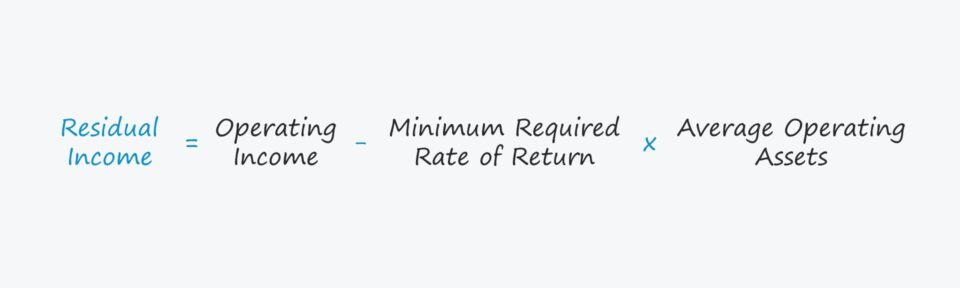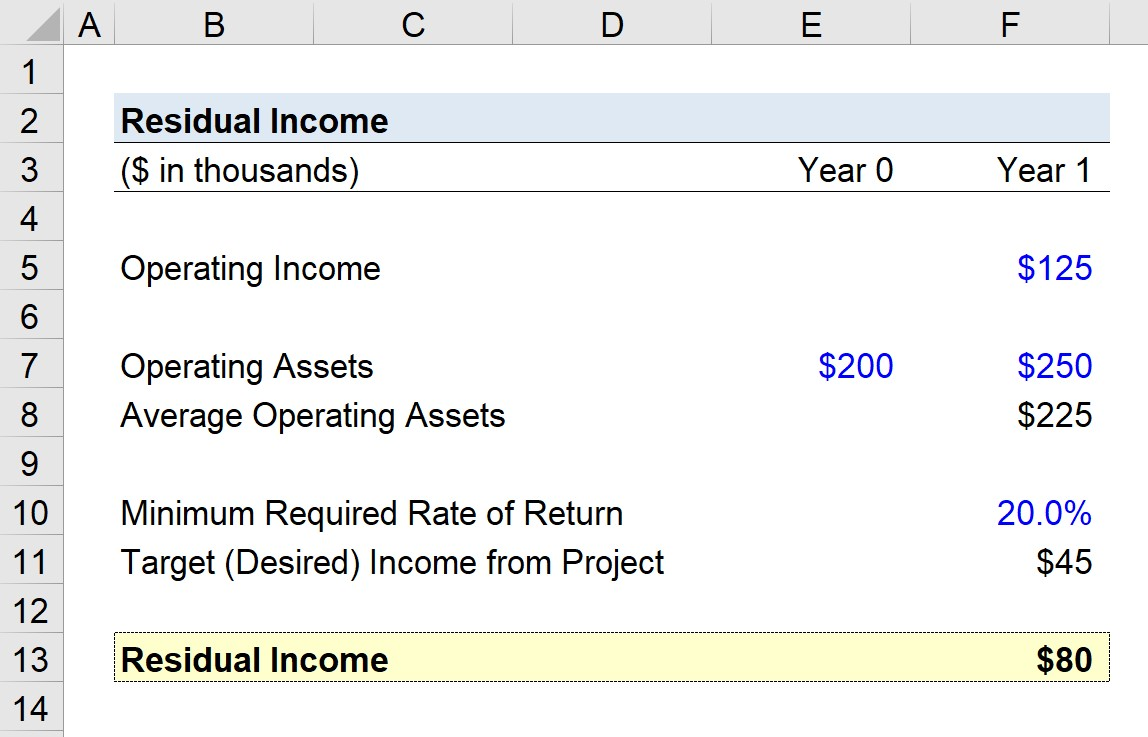Welcome to Wall Street Prep! Use code at checkout for 15% off.# Residual Income (RI)

Guide to Understanding Residual Income## How to Calculate Residual Income (Step-by-Step)

In corporate finance, residual income is defined as the operating income generated by a project or investment in excess of the minimum required rate of return.

The residual income (RI) metric is used by companies to determine whether to pursue certain projects or not.

The first step in estimating the residual income is calculating the product of the minimum required rate of return and the average operating assets.

The minimum required rate of return is conceptually the same as the cost of capital, i.e. the expected return given the risk profile of the project or investment in question.

The minimum return can differ based on the department or division undertaking the project – or be separately estimated based on the operating assets – but the company’s cost of capital can also be used, as it is usually sufficient for general capital budgeting purposes.

From there, the product of the minimum required rate of return and average operating assets is subtracted from the project’s operating income.

## Residual Income Formula

The formula for calculating the residual income is as follows.

Residual Income = Operating Income(Minimum Required Rate of Return × Average Operating Assets)

The product of the minimum required rate of return and average operating assets represents the minimum target return, i.e. the “desired income”.

Target (Desired) Income = Minimum Required Rate of Return × Average Operating Assets

## What is a Good Residual Income?

For purposes of decision-making under the context of capital budgeting, the general rule is to accept a project if the implied residual income is greater than zero.

• If Residual Income > 0 → Accept Project
• If Residual Income < 0 → Reject Project

The generalized rule in capital budgeting states that in order for a company to maximize its firm value, only projects that earn more than the company’s cost of capital should be pursued.

Otherwise, the project will reduce the value of the company rather than create value.

By estimating the residual income before taking on projects, companies can allocate their capital on hand more efficiently to ensure that the return (or potential return) is worth the trade-off in terms of risk.

• Positive Residual Income → Exceeds Minimum Rate of Return
• Negative Residual Income → Lower than Minimum Rate of Return

Of course, the metric will not dictate corporate decisions on its own, but projects with positive residual income are more likely to be accepted internally because of the increased economic incentive.

## Residual Income Calculator

We’ll now move to a modeling exercise, which you can access by filling out the form below.Submitting...

## 1. Project Income and Operating Assets Assumptions

Suppose a company is attempting to decide whether to pursue a project or pass on the opportunity.

The project is projected to generate \$125k in operating income in Year 1.

The value of the operating assets at the beginning of the period (Year 0) was \$200k, while the value was \$250k at the end of the period (Year 1).

• Beginning Operating Assets = \$200k
• Ending Operating Assets = \$250k

By adding those two figures and dividing them by two, the average operating assets equal \$225k.

• Average Operating Assets = \$225k

## 2. Residual Income Calculation Example

If we assume the minimum required rate of return is 20%, what is the project’s residual income?

To determine the project’s residual income, we’ll start by multiplying the minimum required rate of return (20%) by the average operating assets (\$225k).

As mentioned earlier, the resulting amount – \$45k in our example – represents the target (desired) income from the project.

The more excess income there is above the target (desired) income, the more profitable the project is.

The final step is to subtract the target (desired) income amount from the project’s operating income (\$125k).

The resulting figure is \$80k, which represents the project’s residual income. Because this figure is positive, it suggests the project should likely be approved.

• Residual Income = \$125k – (20% × \$225k) = \$80kStep-by-Step Online Course

### Everything You Need To Master Financial Modeling

Enroll in The Premium Package: Learn Financial Statement Modeling, DCF, M&A, LBO and Comps. The same training program used at top investment banks.Inline FeedbacksFebruary 26, 2023 4:25 pmFebruary 28, 2023 3:36 pm